# NCERT Exemplar Solutions for Class 9 Maths Chapter 8 - Quadrilaterals

## NCERT Exemplar Solutions Class 9 Maths Chapter 8 – Free PDF Download

NCERT Exemplar Class 9 Mathematics Chapter 8 Quadrilaterals, is provided here for students to prepare for exams and score good marks. These NCERT Exemplar Class 9 Maths problems have been designed according to the CBSE syllabus (2021-2022) for 9th standard by our experts, which covers the following topics of chapter Quadrilaterals given below:

• Angle sum property of quadrilaterals and it’s proof
• Types of quadrilaterals such as Trapezium, Parallelogram, Square, Rectangle, Rhombus, and Kite.
• Properties of parallelogram and condition for a quadrilateral to be a parallelogram
• Problems based on mid-point theorem

A quadrilateral is a polygon that has four vertices and four sides. In this chapter, the students will learn the properties of a parallelogram, and a theorem which is known as midpoint theorem. To master the concepts of quadrilaterals students are advised to solve NCERT Exemplar for Chapter 8 Quadrilaterals.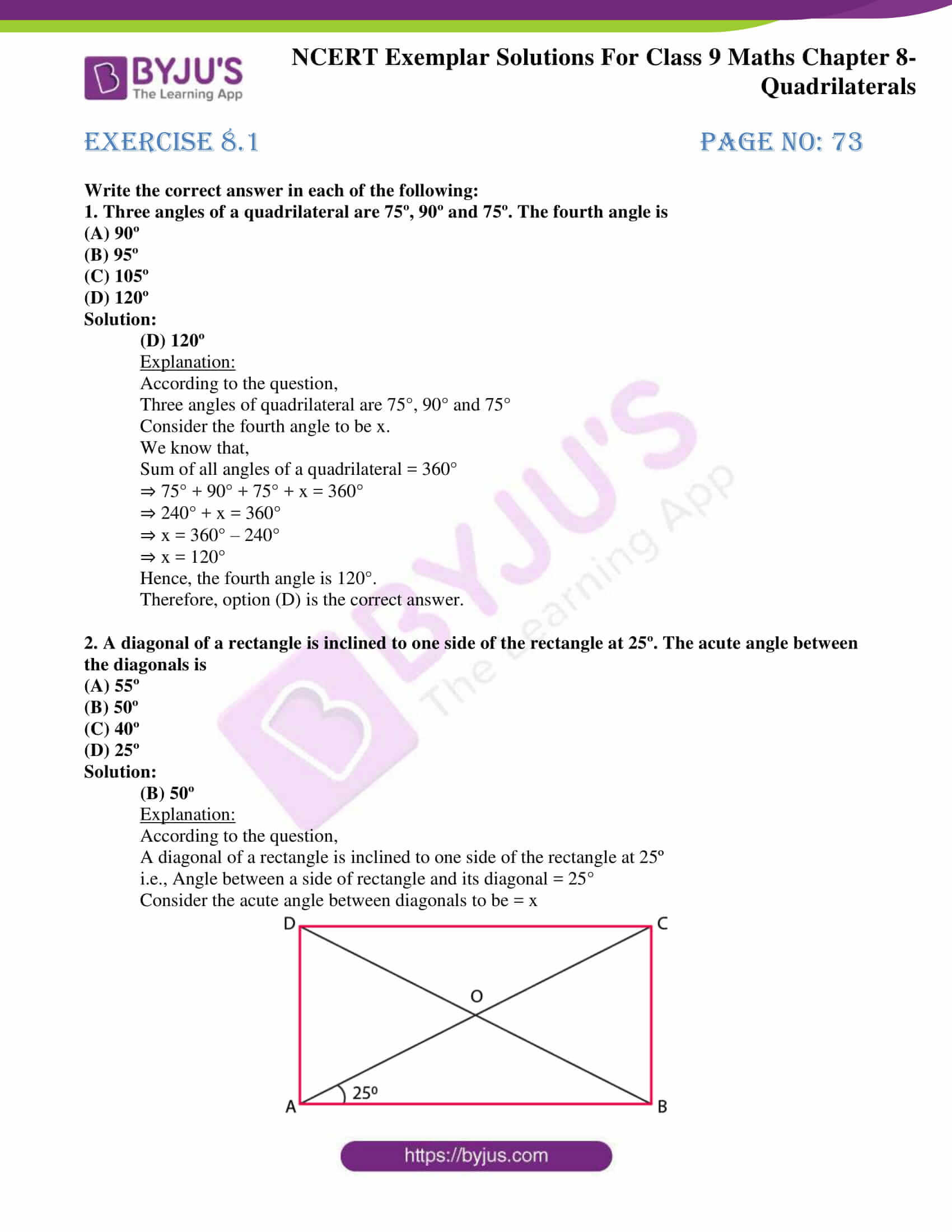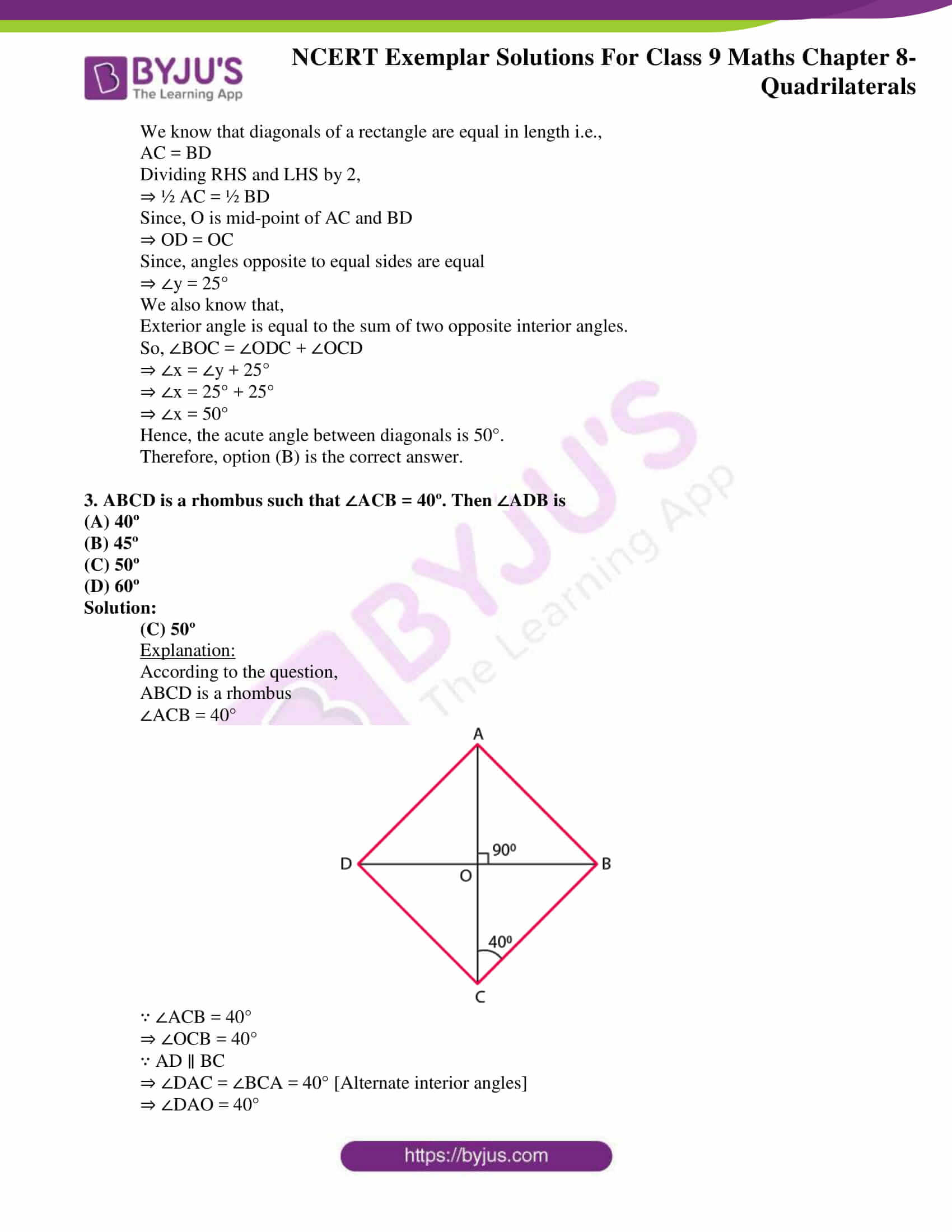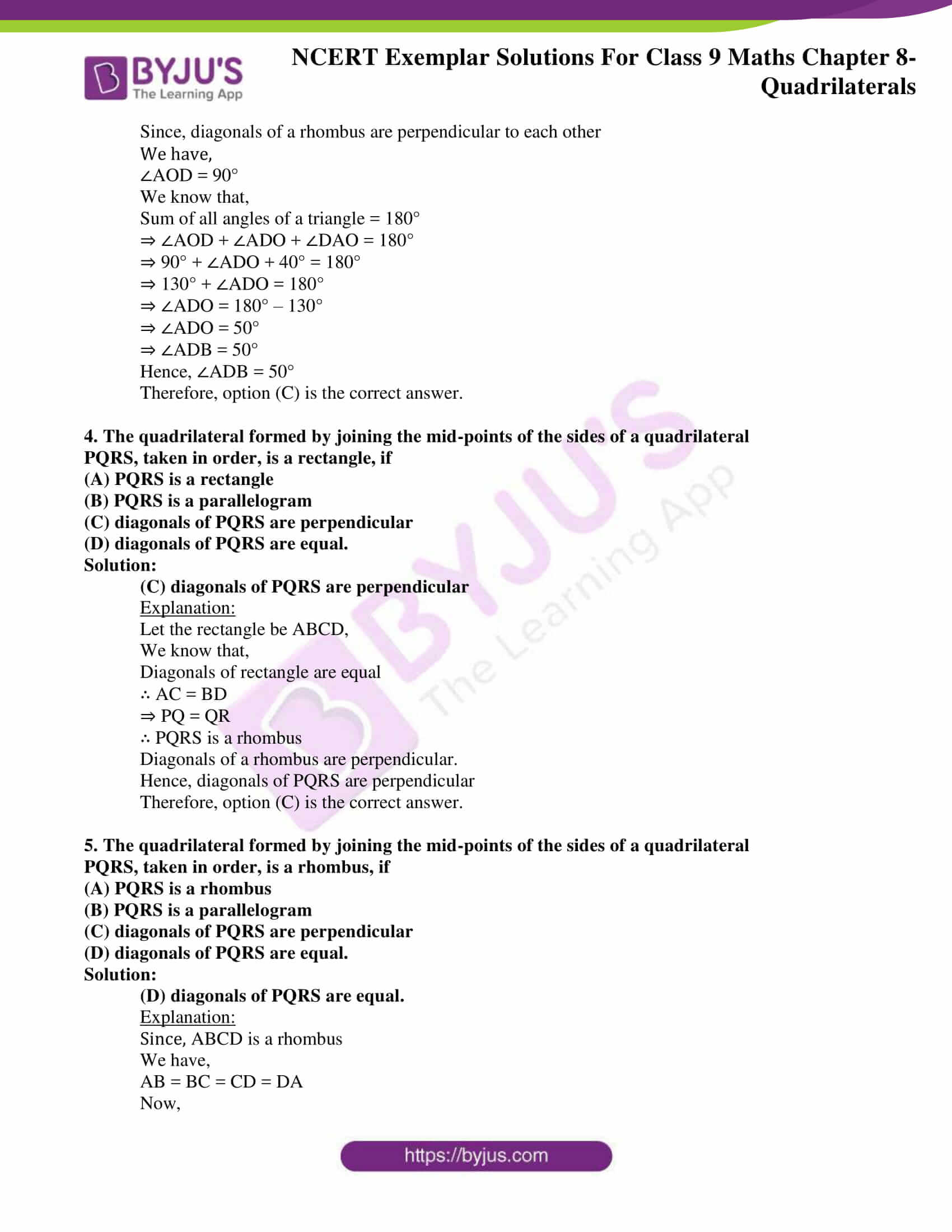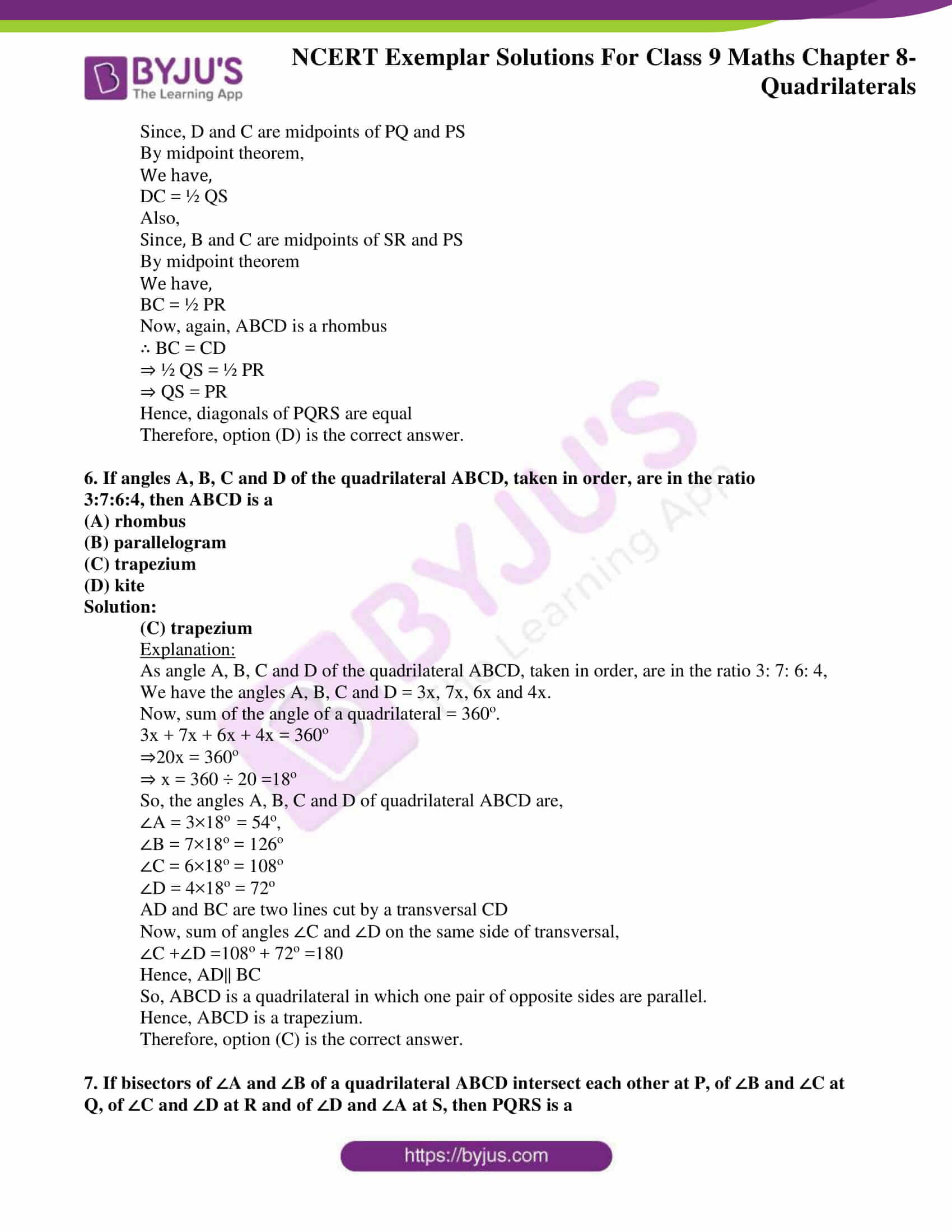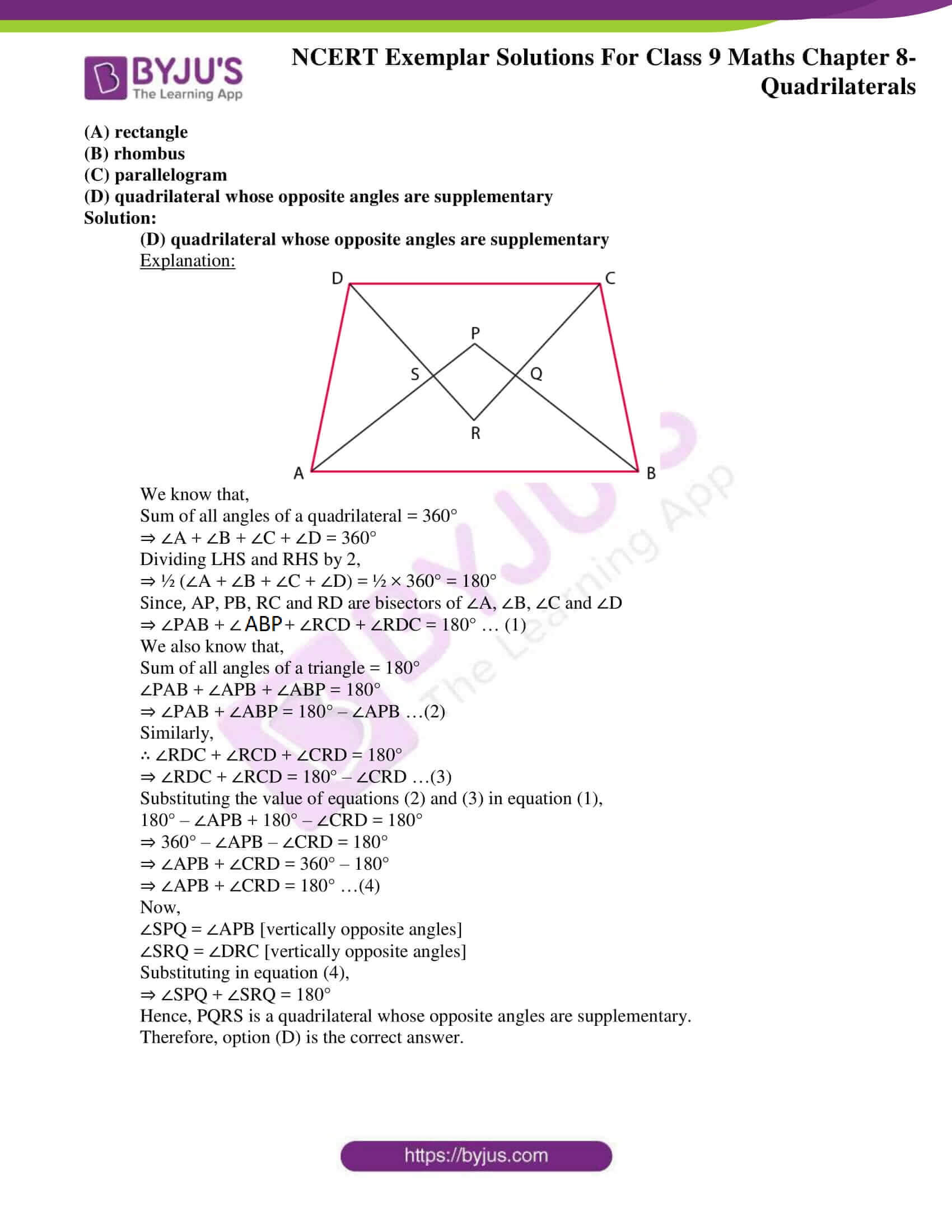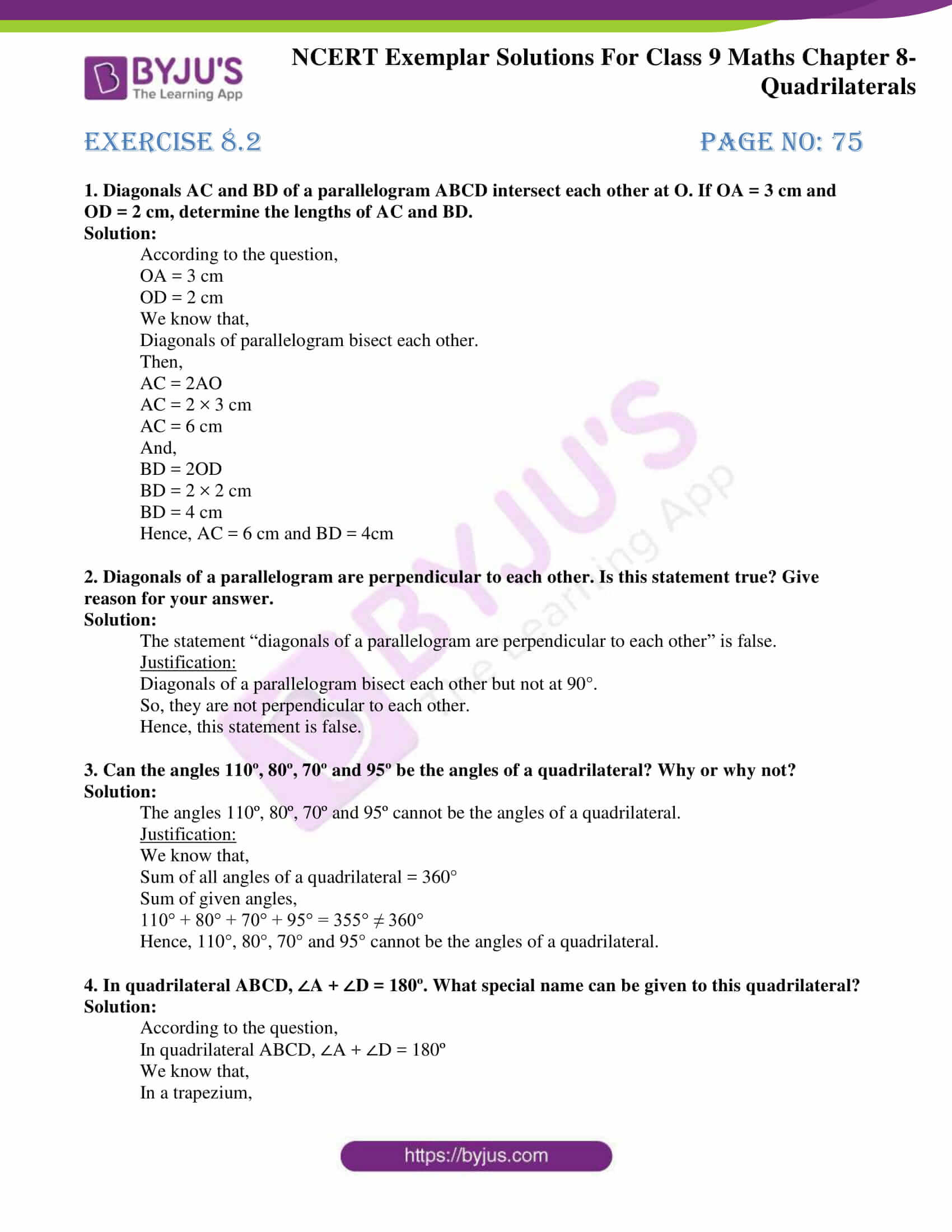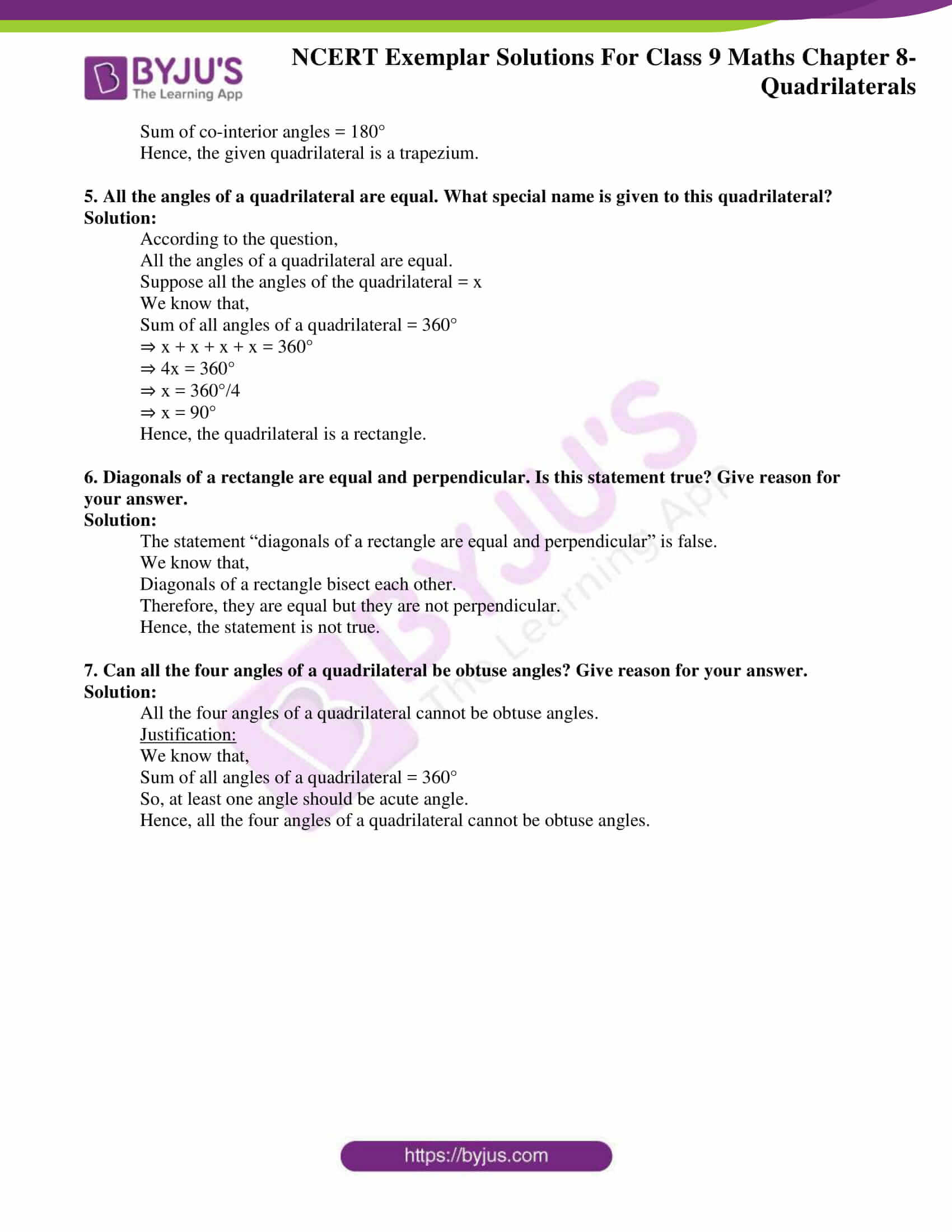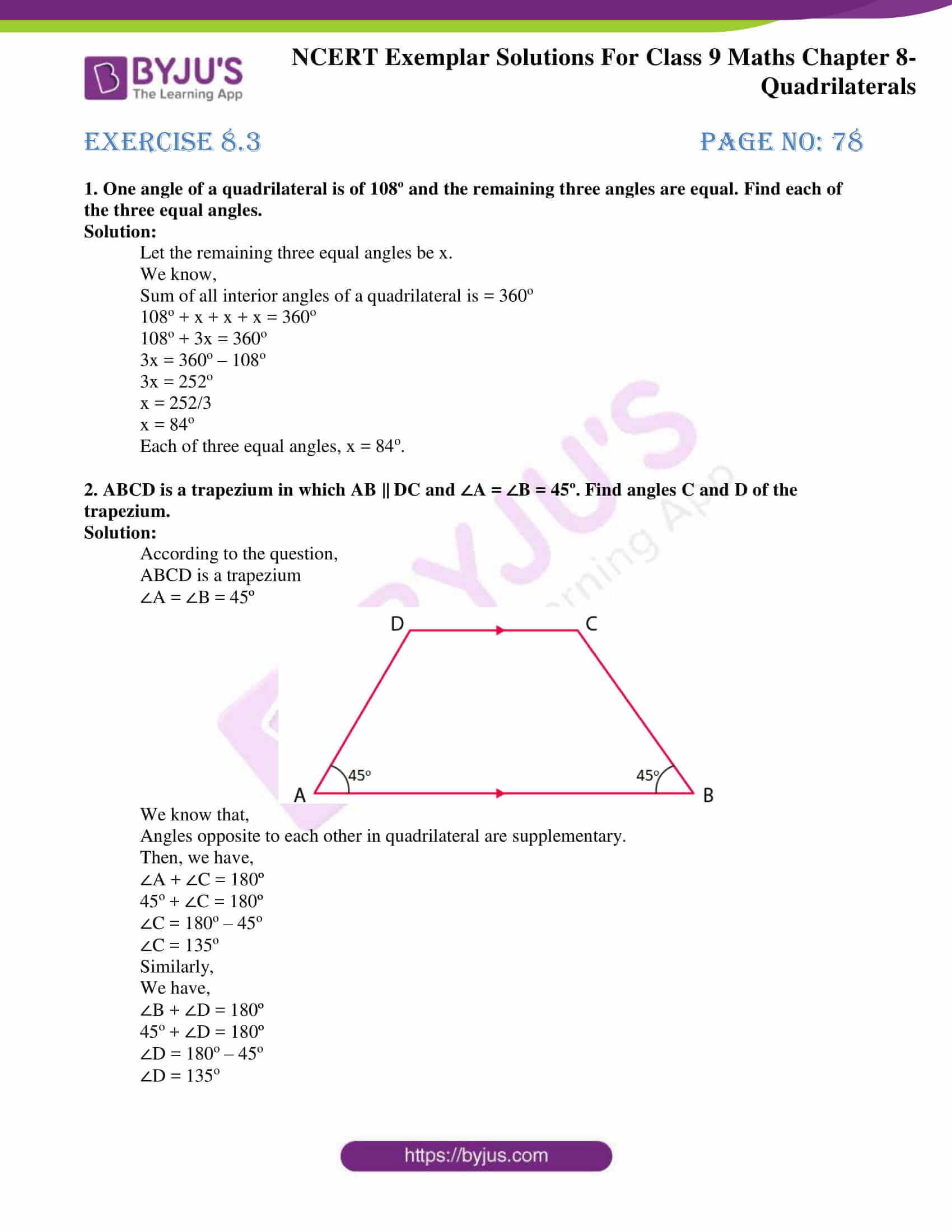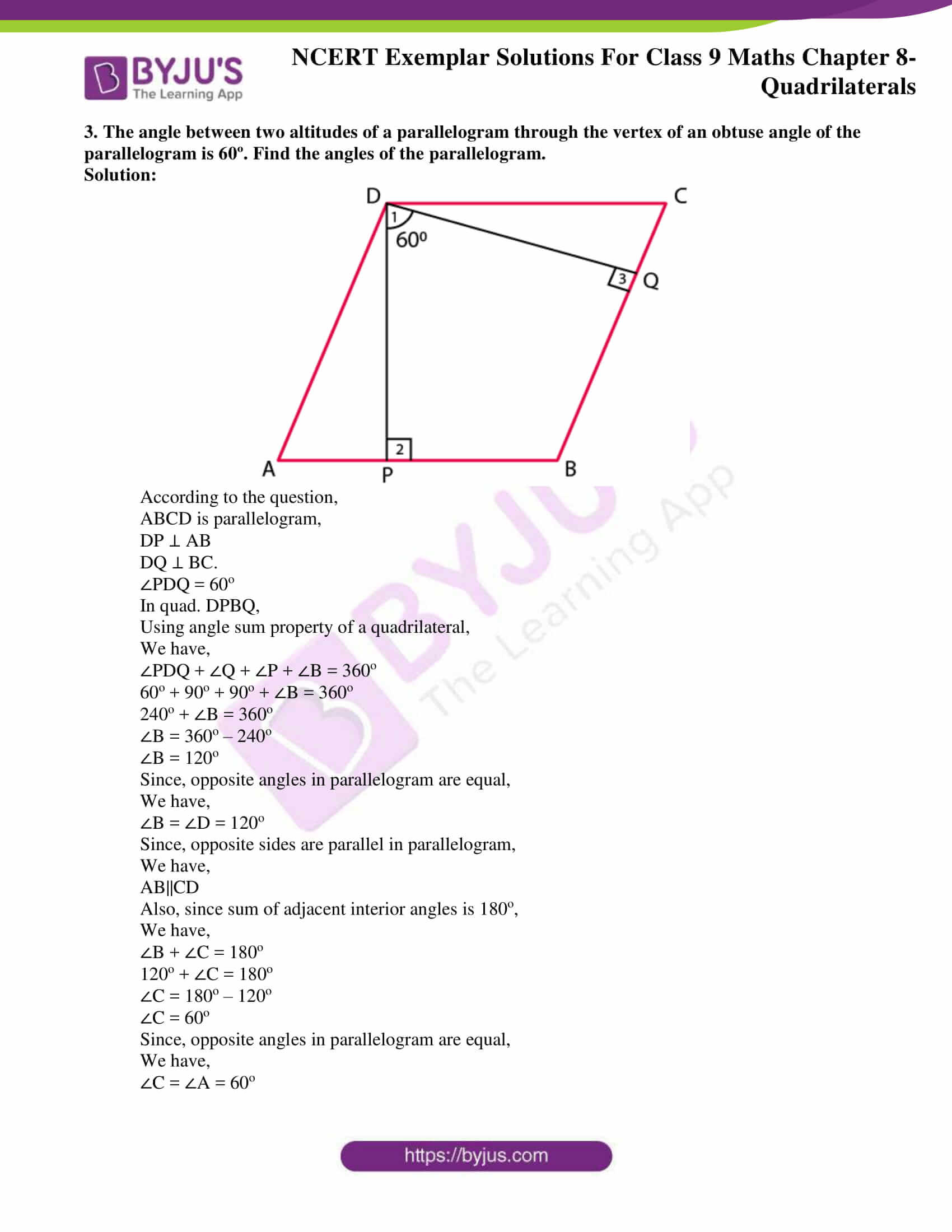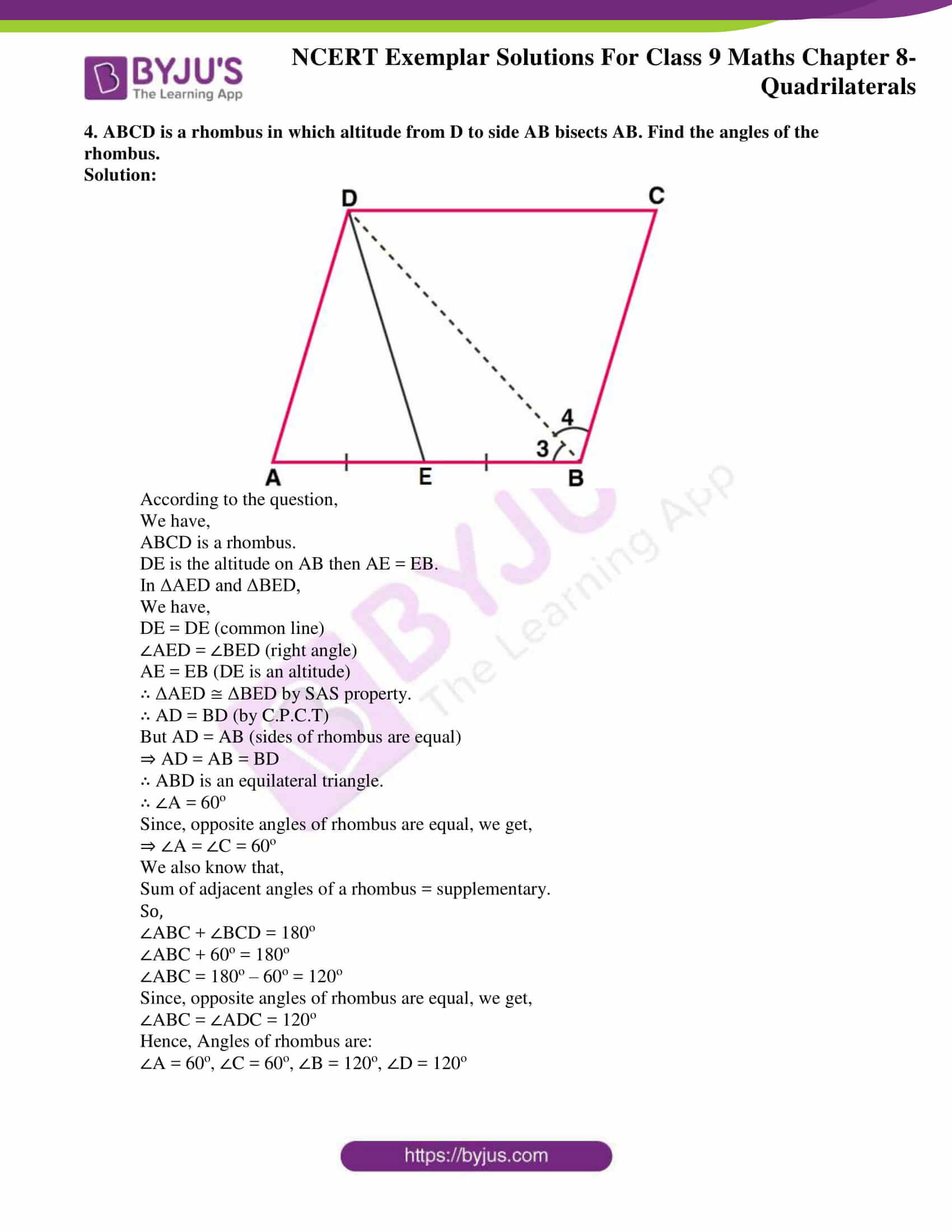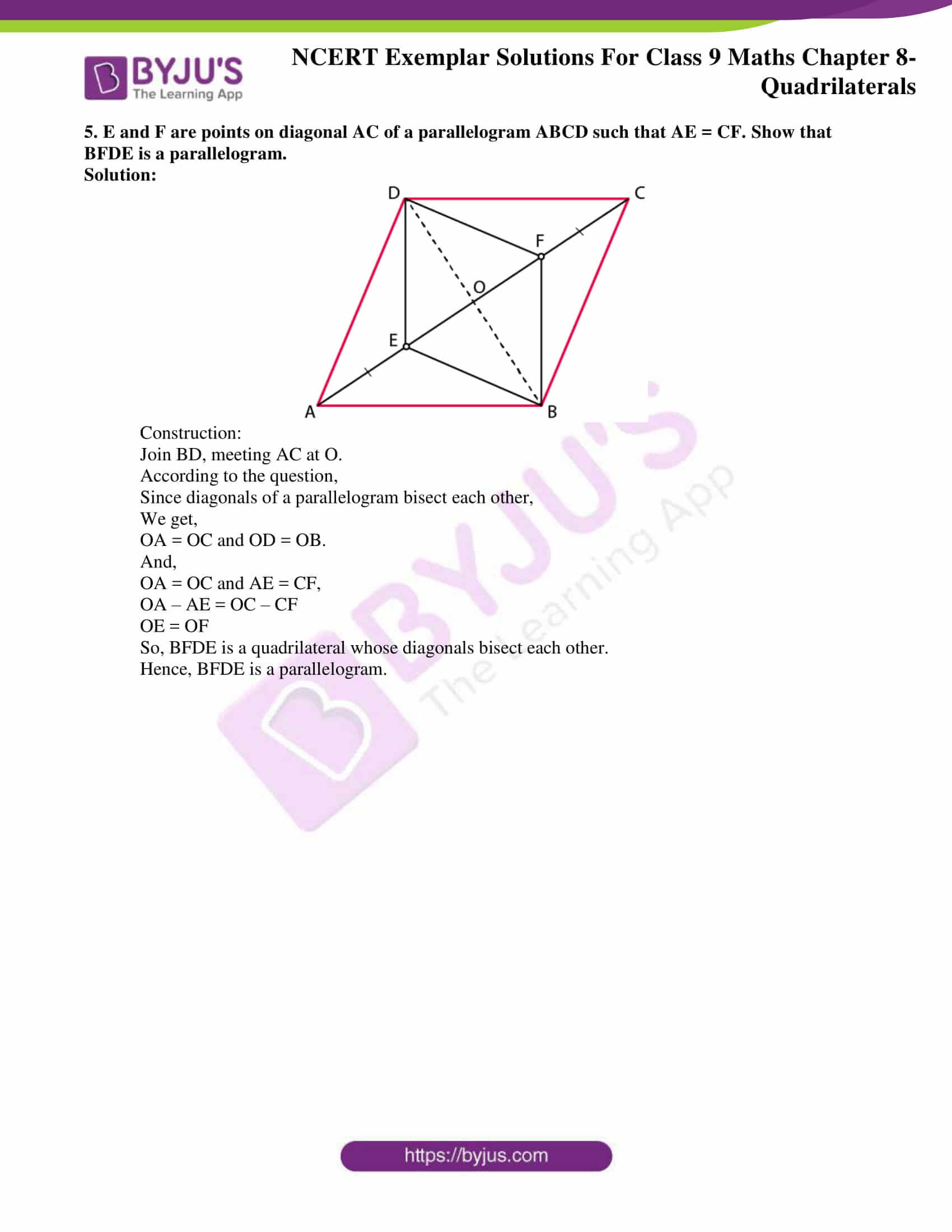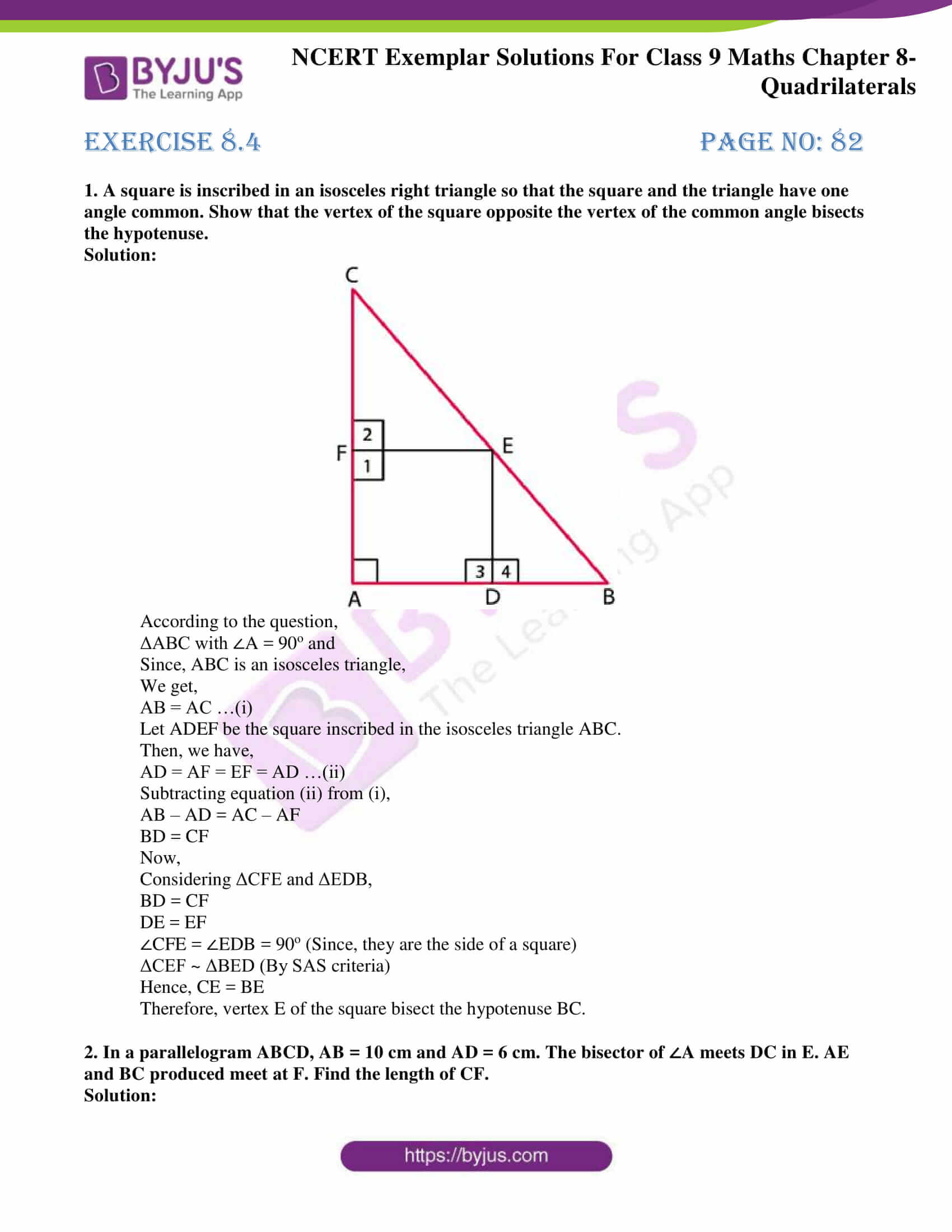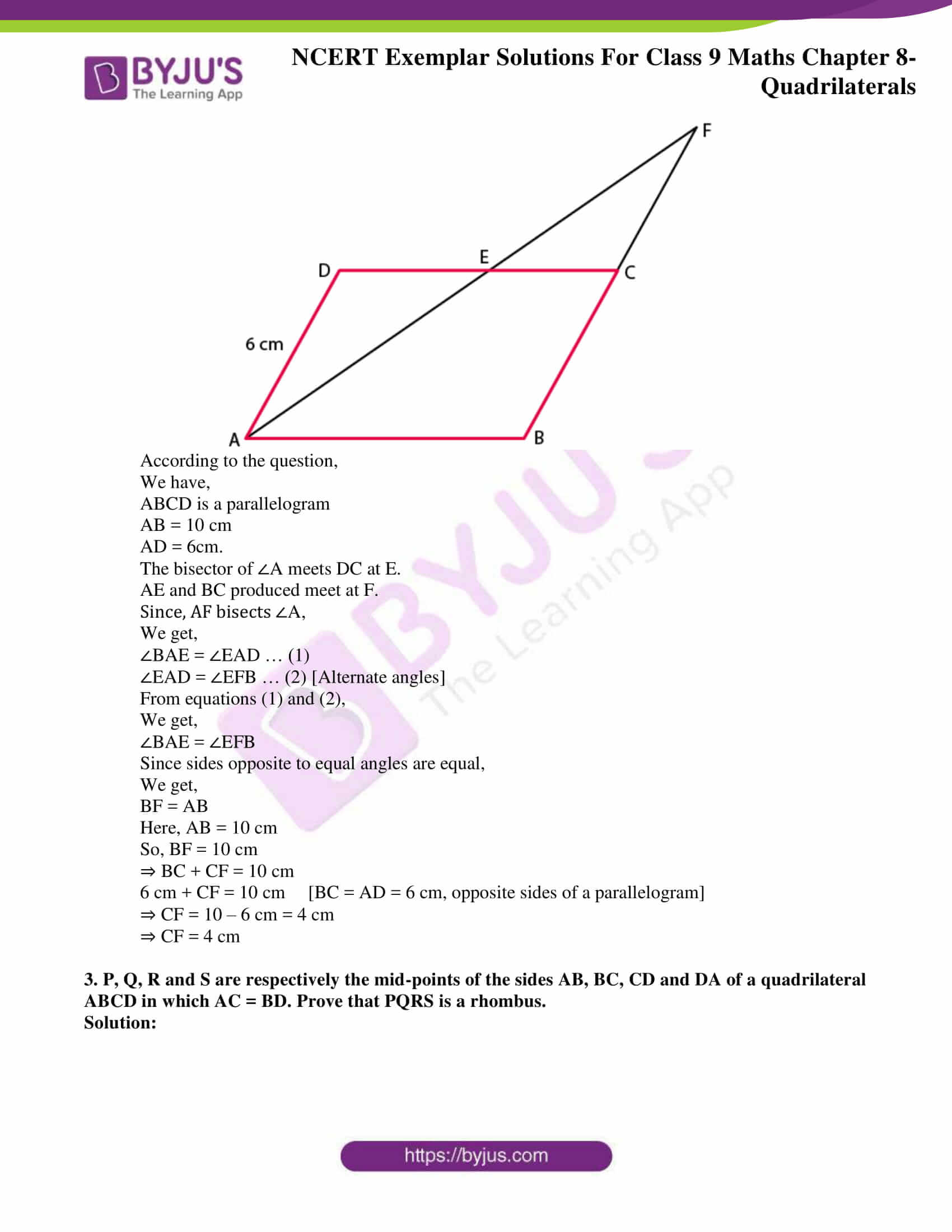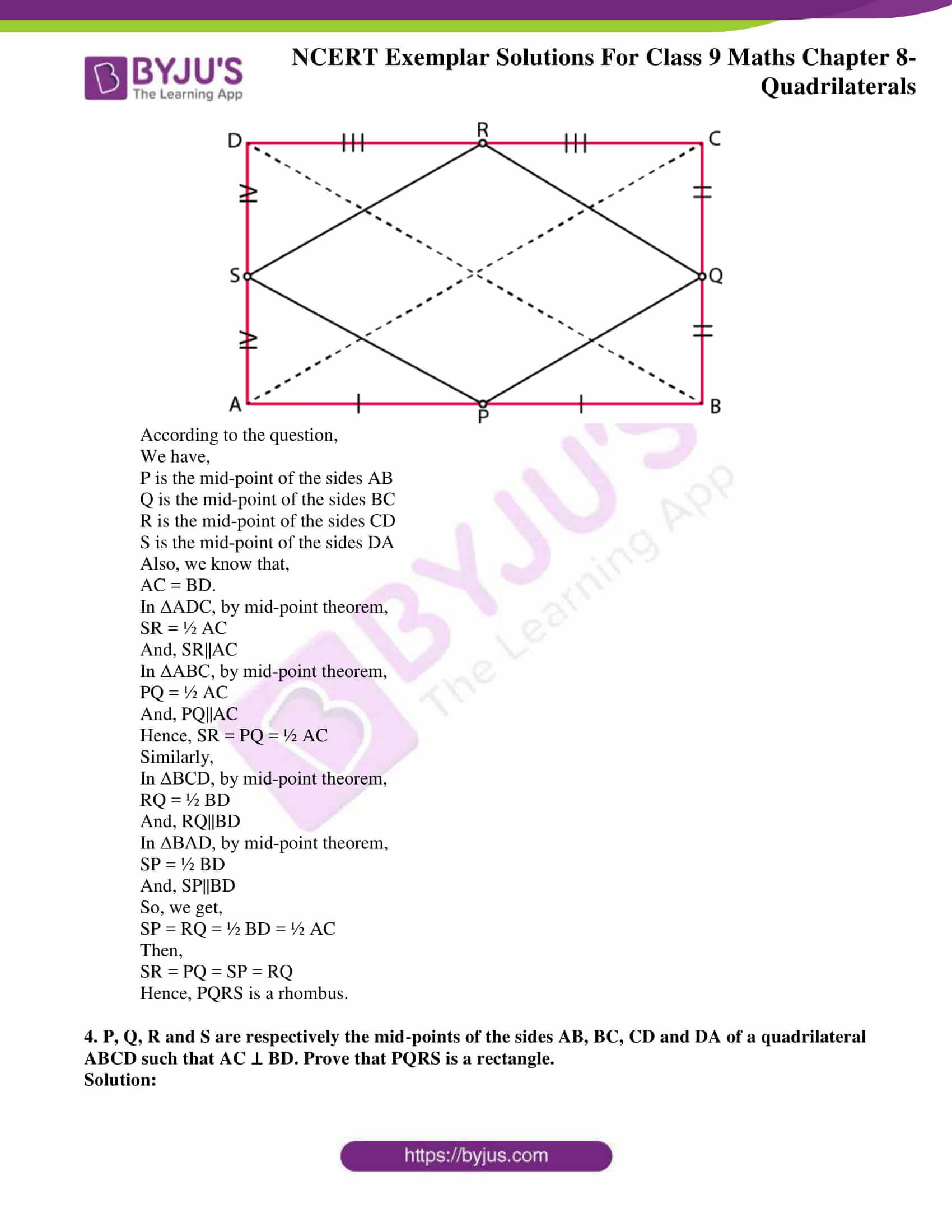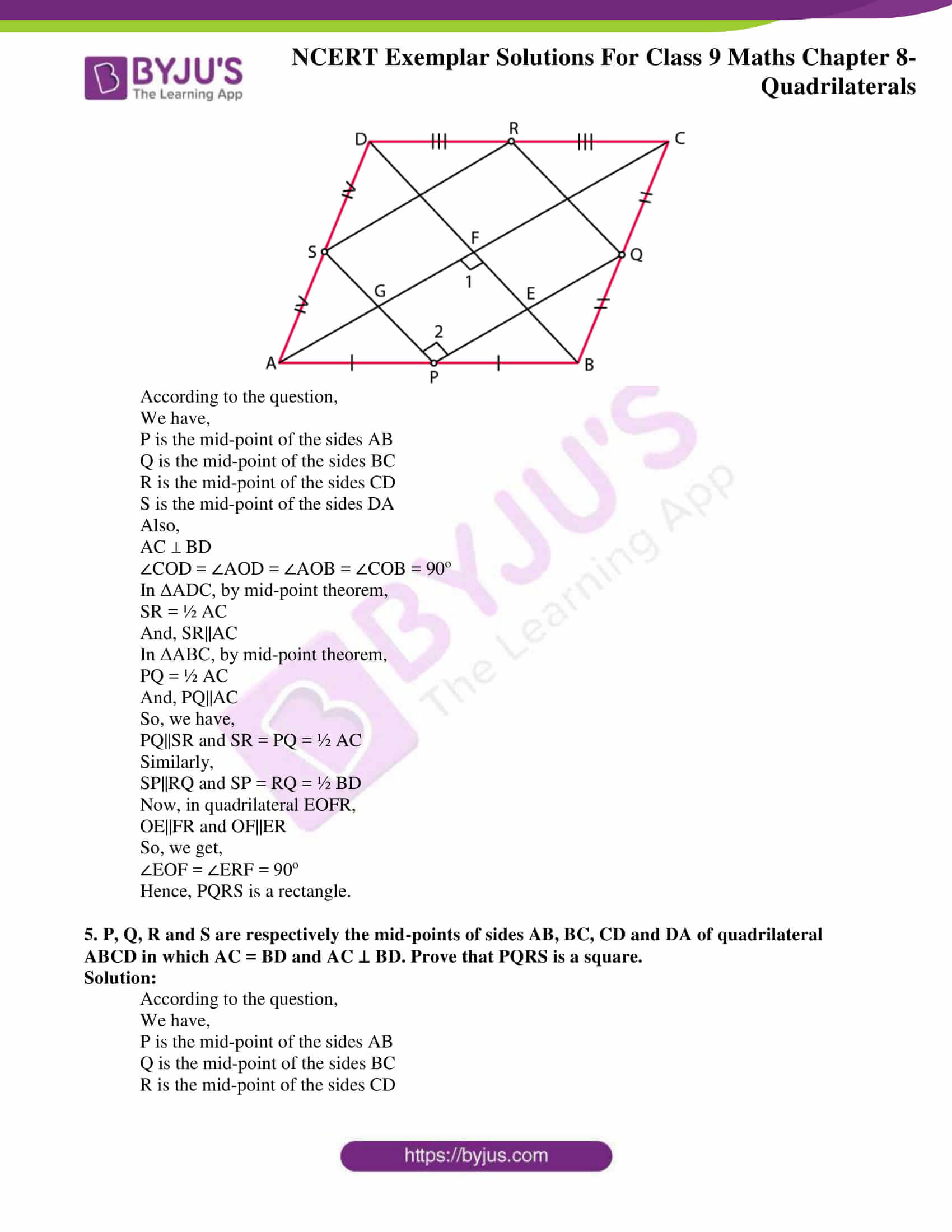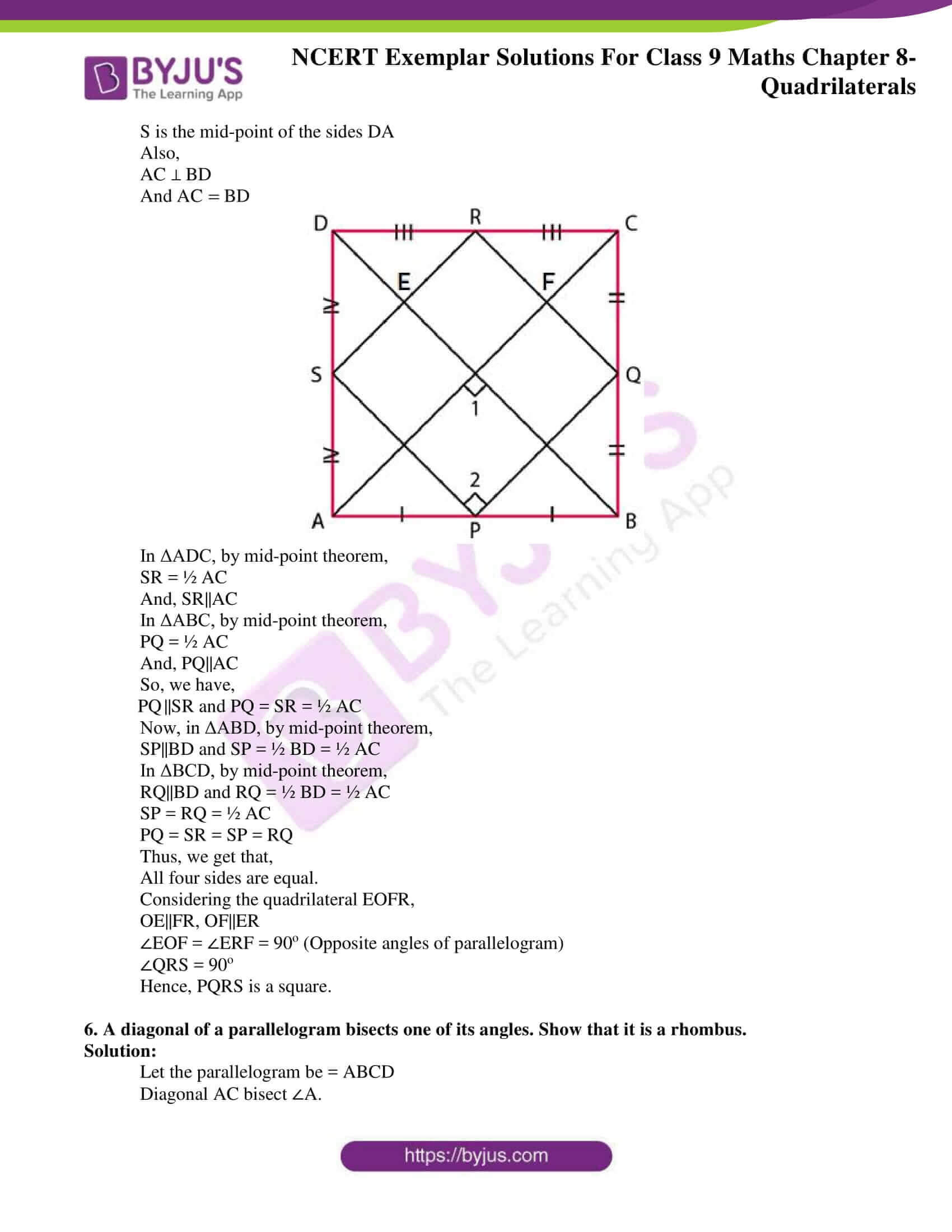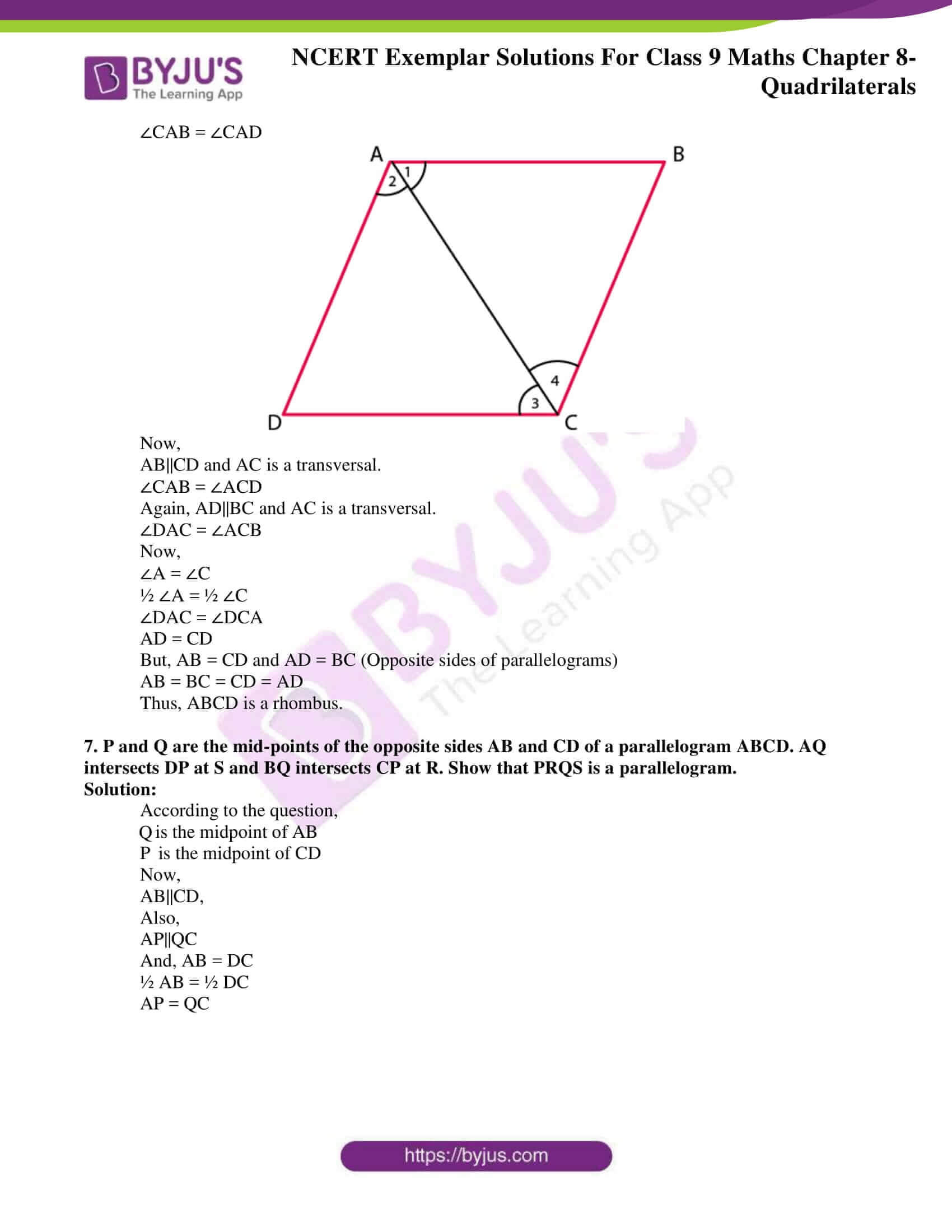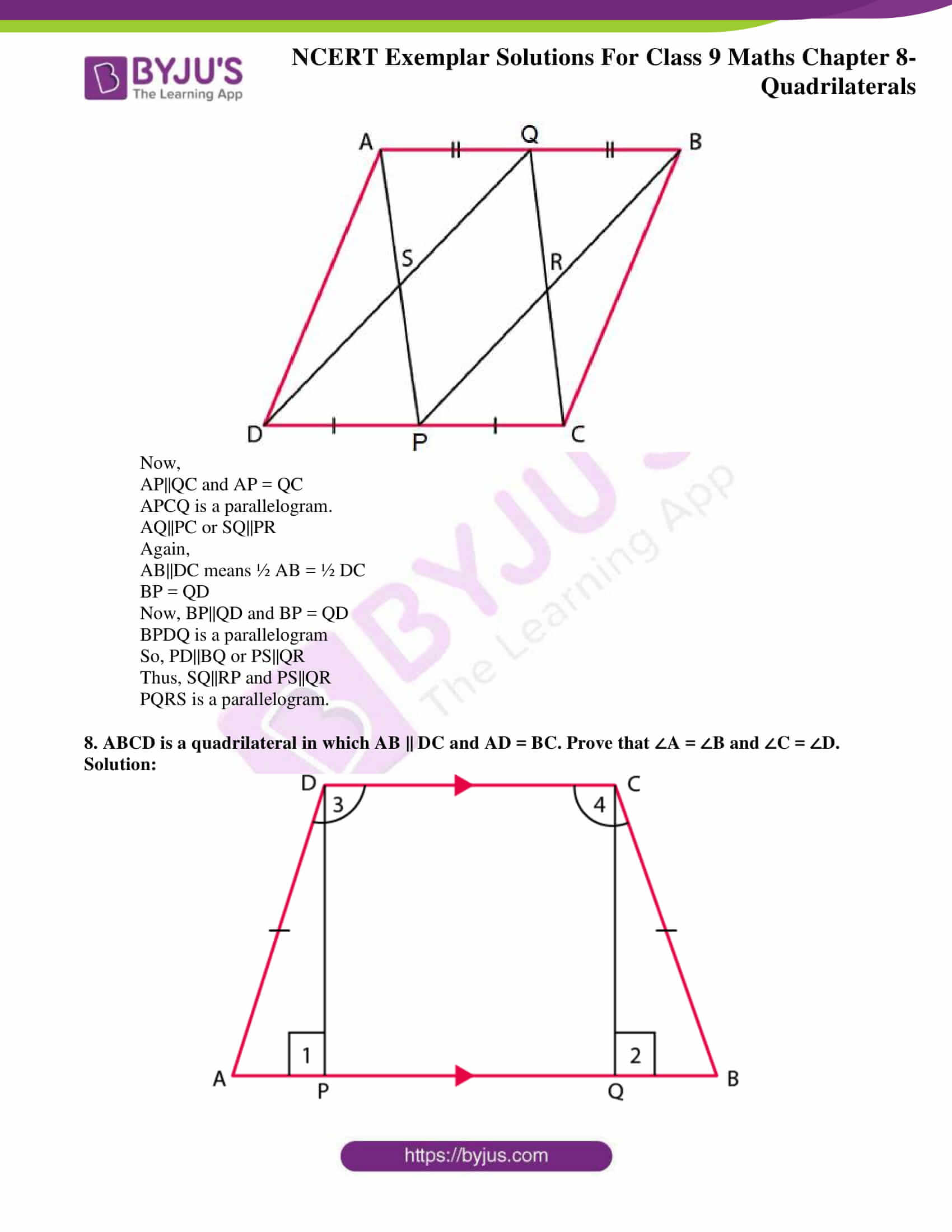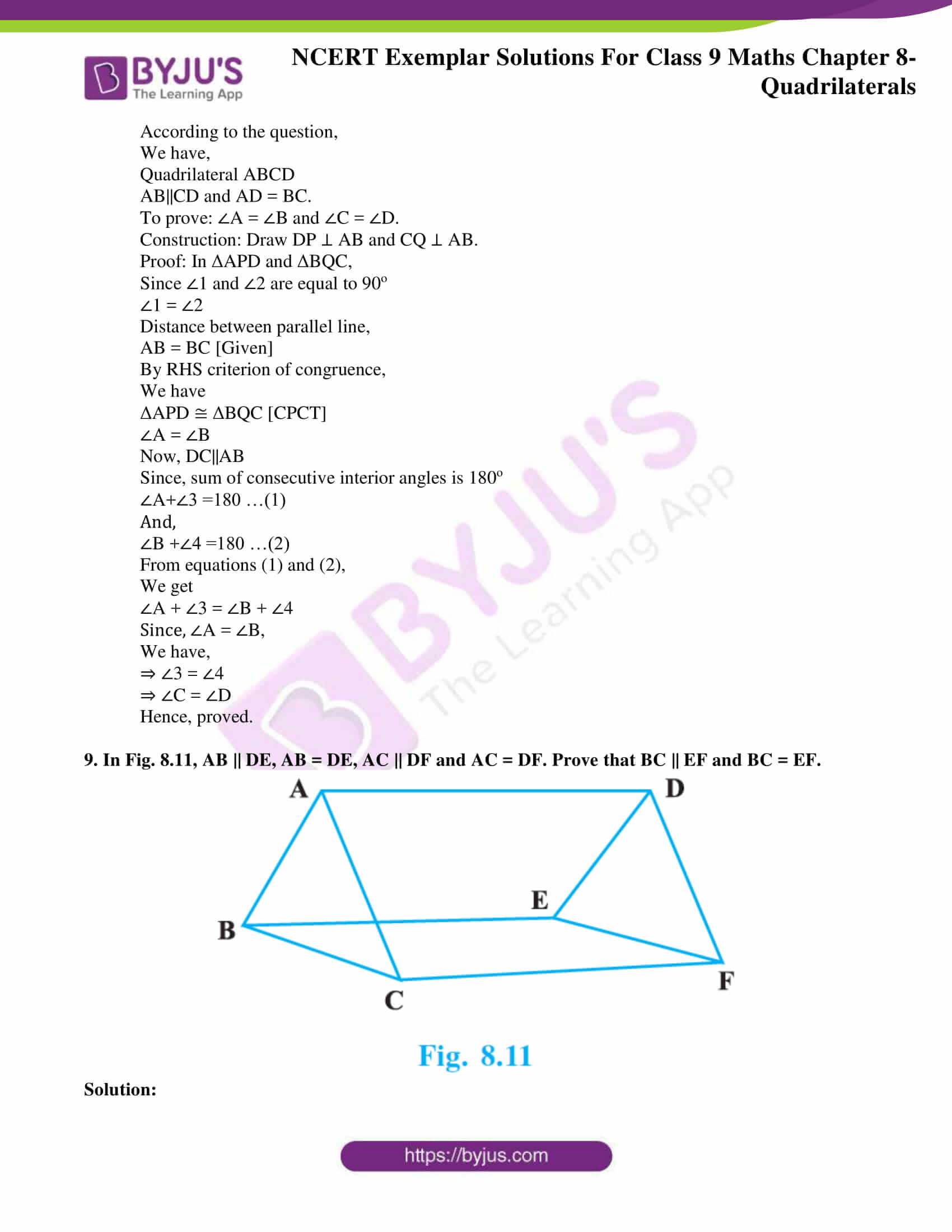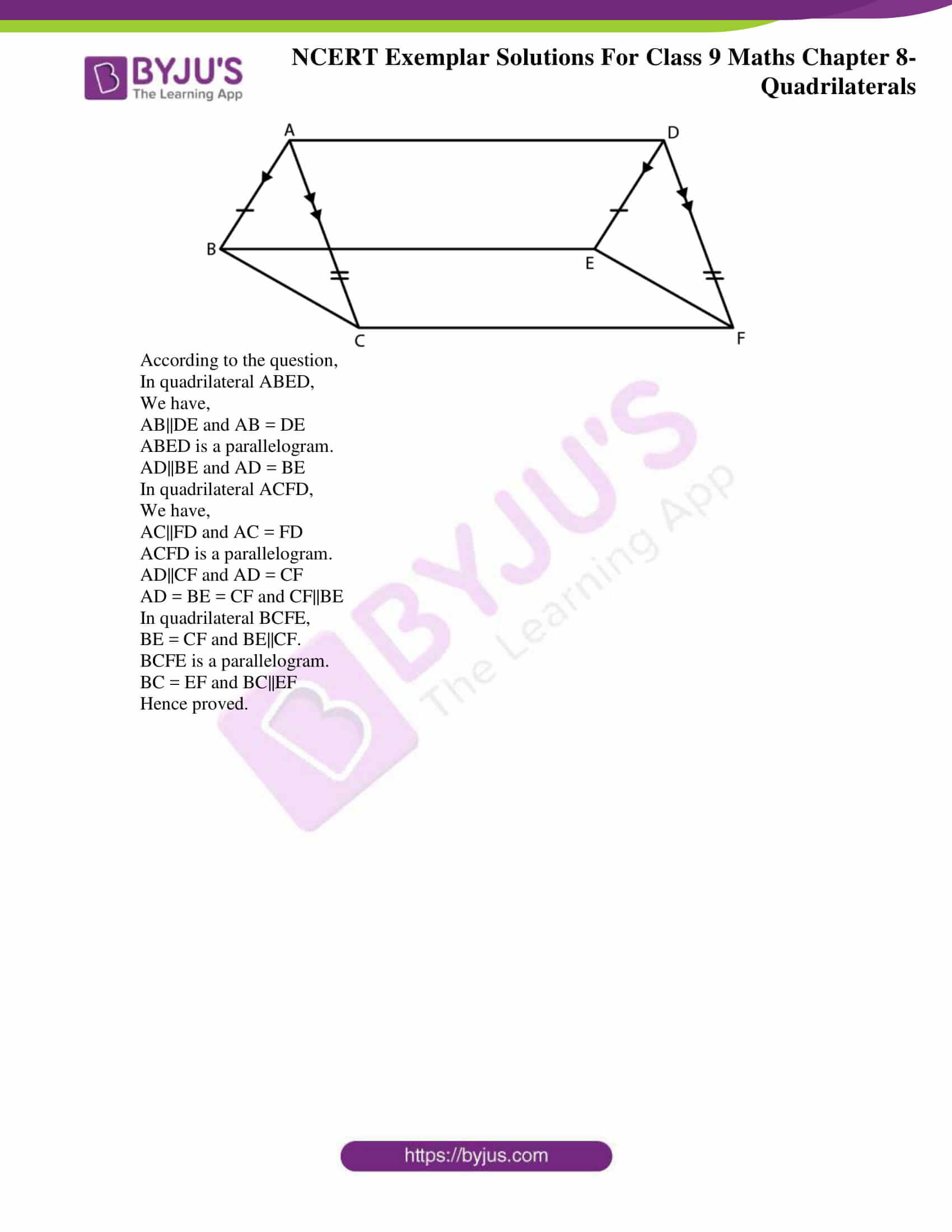### Access Answers to NCERT Exemplar Solutions for Class 9 Maths Chapter 8 Quadrilaterals

Exercise 8.1 Page No: 73

Write the correct answer in each of the following:

1. Three angles of a quadrilateral are 75º, 90º and 75º. The fourth angle is

(A) 90º

(B) 95º

(C) 105º

(D) 120º

Solution:

(D) 120º

Explanation:

According to the question,

Three angles of quadrilateral are 75°, 90° and 75°

Consider the fourth angle to be x.

We know that,

Sum of all angles of a quadrilateral = 360°

⇒ 75° + 90° + 75° + x = 360°

⇒ 240° + x = 360°

⇒ x = 360° – 240°

⇒ x = 120°

Hence, the fourth angle is 120°.

Therefore, option (D) is the correct answer.

2. A diagonal of a rectangle is inclined to one side of the rectangle at 25º. The acute angle between the diagonals is

(A) 55º

(B) 50º

(C) 40º

(D) 25º

Solution:

(B) 50º

Explanation:

According to the question,

A diagonal of a rectangle is inclined to one side of the rectangle at 25º

i.e., Angle between a side of rectangle and its diagonal = 25°

Consider the acute angle between diagonals to be = x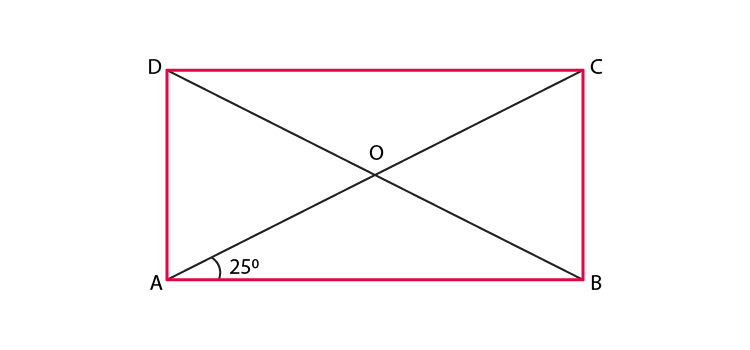We know that diagonals of a rectangle are equal in length i.e.,

AC = BD

Dividing RHS and LHS by 2,

⇒ ½ AC = ½ BD

Since, O is mid-point of AC and BD

⇒ OD = OC

Since, angles opposite to equal sides are equal

⇒ ∠y = 25°

We also know that,

Exterior angle is equal to the sum of two opposite interior angles.

So, ∠BOC = ∠ODC + ∠OCD

⇒ ∠x = ∠y + 25°

⇒ ∠x = 25° + 25°

⇒ ∠x = 50°

Hence, the acute angle between diagonals is 50°.

Therefore, option (B) is the correct answer.

3. ABCD is a rhombus such that ∠ACB = 40º. Then ∠ADB is

(A) 40º

(B) 45º

(C) 50º

(D) 60º

Solution:

(C) 50º

Explanation:

According to the question,

ABCD is a rhombus

∠ACB = 40°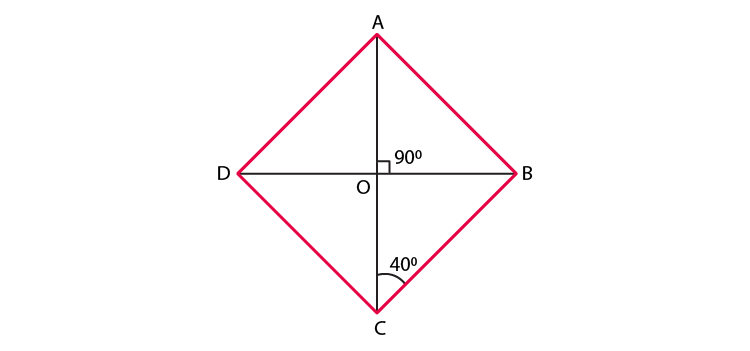∵ ∠ACB = 40°

⇒ ∠OCB = 40°

⇒ ∠DAC = ∠BCA = 40° [Alternate interior angles]

⇒ ∠DAO = 40°

Since, diagonals of a rhombus are perpendicular to each other

We have,

∠AOD = 90°

We know that,

Sum of all angles of a triangle = 180°

⇒ ∠AOD + ∠ADO + ∠DAO = 180°

⇒ 90° + ∠ADO + 40° = 180°

⇒ 130° + ∠ADO = 180°

⇒ ∠ADO = 180° – 130°

Therefore, option (C) is the correct answer.

4. The quadrilateral formed by joining the mid-points of the sides of a quadrilateral

PQRS, taken in order, is a rectangle, if

(A) PQRS is a rectangle

(B) PQRS is a parallelogram

(C) diagonals of PQRS are perpendicular

(D) diagonals of PQRS are equal.

Solution:

(C) diagonals of PQRS are perpendicular

Explanation:

Let the rectangle be ABCD,

We know that,

Diagonals of rectangle are equal

∴ AC = BD

⇒ PQ = QR

∴ PQRS is a rhombus

Diagonals of a rhombus are perpendicular.

Hence, diagonals of PQRS are perpendicular

Therefore, option (C) is the correct answer.

5. The quadrilateral formed by joining the mid-points of the sides of a quadrilateral

PQRS, taken in order, is a rhombus, if

(A) PQRS is a rhombus

(B) PQRS is a parallelogram

(C) diagonals of PQRS are perpendicular

(D) diagonals of PQRS are equal.

Solution:

(D) diagonals of PQRS are equal.

Explanation:

Since, ABCD is a rhombus

We have,

AB = BC = CD = DA

Now,

Since, D and C are midpoints of PQ and PS

By midpoint theorem,

We have,

DC = ½ QS

Also,

Since, B and C are midpoints of SR and PS

By midpoint theorem

We have,

BC = ½ PR

Now, again, ABCD is a rhombus

∴ BC = CD

⇒ ½ QS = ½ PR

⇒ QS = PR

Hence, diagonals of PQRS are equal

Therefore, option (D) is the correct answer.

6. If angles A, B, C and D of the quadrilateral ABCD, taken in order, are in the ratio

3:7:6:4, then ABCD is a

(A) rhombus

(B) parallelogram

(C) trapezium

(D) kite

Solution:

(C) trapezium

Explanation:

As angle A, B, C and D of the quadrilateral ABCD, taken in order, are in the ratio 3: 7: 6: 4,

We have the angles A, B, C and D = 3x, 7x, 6x and 4x.

Now, sum of the angle of a quadrilateral = 360o.

3x + 7x + 6x + 4x = 360o

⇒20x = 360o

⇒ x = 360 ÷ 20 =18o

So, the angles A, B, C and D of quadrilateral ABCD are,

∠A = 3×18o = 54o,

∠B = 7×18o = 126o

∠C = 6×18o = 108o

∠D = 4×18o = 72o

AD and BC are two lines cut by a transversal CD

Now, sum of angles ∠C and ∠D on the same side of transversal,

∠C +∠D =108o + 72o =180

So, ABCD is a quadrilateral in which one pair of opposite sides are parallel.

Hence, ABCD is a trapezium.

Therefore, option (C) is the correct answer.

7. If bisectors of ∠A and ∠B of a quadrilateral ABCD intersect each other at P, of ∠B and ∠C at Q, of ∠C and ∠D at R and of ∠D and ∠A at S, then PQRS is a

(A) rectangle

(B) rhombus

(C) parallelogram

(D) quadrilateral whose opposite angles are supplementary

Solution:

(D) quadrilateral whose opposite angles are supplementary

Explanation: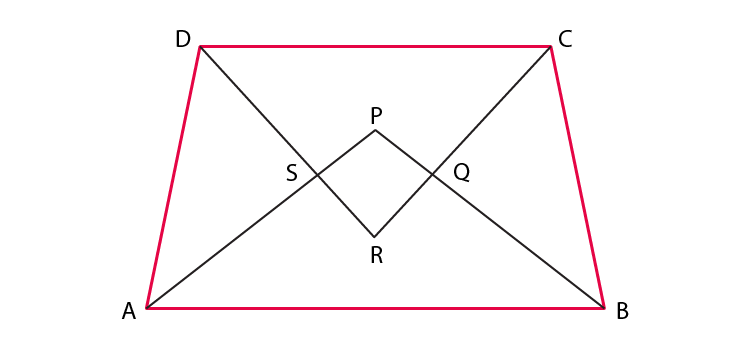We know that,

Sum of all angles of a quadrilateral = 360°

⇒ ∠A + ∠B + ∠C + ∠D = 360°

Dividing LHS and RHS by 2,

⇒ ½ (∠A + ∠B + ∠C + ∠D) = ½ × 360° = 180°

Since, AP, PB, RC and RD are bisectors of ∠A, ∠B, ∠C and ∠D

⇒ ∠PAB + ∠ABP + ∠RCD + ∠RDC = 180° … (1)

We also know that,

Sum of all angles of a triangle = 180°

∠PAB + ∠APB + ∠ABP = 180°

⇒ ∠PAB + ∠ABP = 180° – ∠APB …(2)

Similarly,

∴ ∠RDC + ∠RCD + ∠CRD = 180°

⇒ ∠RDC + ∠RCD = 180° – ∠CRD …(3)

Substituting the value of equations (2) and (3) in equation (1),

180° – ∠APB + 180° – ∠CRD = 180°

⇒ 360° – ∠APB – ∠CRD = 180°

⇒ ∠APB + ∠CRD = 360° – 180°

⇒ ∠APB + ∠CRD = 180° …(4)

Now,

∠SPQ = ∠APB [vertically opposite angles]

∠SRQ = ∠DRC [vertically opposite angles]

Substituting in equation (4),

⇒ ∠SPQ + ∠SRQ = 180°

Hence, PQRS is a quadrilateral whose opposite angles are supplementary.

Therefore, option (D) is the correct answer.

Exercise 8.2 Page No: 75

1. Diagonals AC and BD of a parallelogram ABCD intersect each other at O. If OA = 3 cm and OD = 2 cm, determine the lengths of AC and BD.

Solution:

According to the question,

OA = 3 cm

OD = 2 cm

We know that,

Diagonals of parallelogram bisect each other.

Then,

AC = 2AO

AC = 2 × 3 cm

AC = 6 cm

And,

BD = 2OD

BD = 2 × 2 cm

BD = 4 cm

Hence, AC = 6 cm and BD = 4cm

2. Diagonals of a parallelogram are perpendicular to each other. Is this statement true? Give reason for your answer.

Solution:

The statement “diagonals of a parallelogram are perpendicular to each other” is false.

Justification:

Diagonals of a parallelogram bisect each other but not at 90°.

So, they are not perpendicular to each other.

Hence, this statement is false.

3. Can the angles 110º, 80º, 70º and 95º be the angles of a quadrilateral? Why or why not?

Solution:

The angles 110º, 80º, 70º and 95º cannot be the angles of a quadrilateral.

Justification:

We know that,

Sum of all angles of a quadrilateral = 360°

Sum of given angles,

110° + 80° + 70° + 95° = 355° ≠ 360°

Hence, 110°, 80°, 70° and 95° cannot be the angles of a quadrilateral.

4. In quadrilateral ABCD, ∠A + ∠D = 180º. What special name can be given to this quadrilateral?

Solution:

According to the question,

In quadrilateral ABCD, ∠A + ∠D = 180º

We know that,

In a trapezium,

Sum of co-interior angles = 180°

Hence, the given quadrilateral is a trapezium.

5. All the angles of a quadrilateral are equal. What special name is given to this quadrilateral?

Solution:

According to the question,

All the angles of a quadrilateral are equal.

Suppose all the angles of the quadrilateral = x

We know that,

Sum of all angles of a quadrilateral = 360°

⇒ x + x + x + x = 360°

⇒ 4x = 360°

⇒ x = 360°/4

⇒ x = 90°

Hence, the quadrilateral is a rectangle.

6. Diagonals of a rectangle are equal and perpendicular. Is this statement true? Give reason for your answer.

Solution:

The statement “diagonals of a rectangle are equal and perpendicular” is false.

We know that,

Diagonals of a rectangle bisect each other.

Therefore, they are equal but they are not perpendicular.

Hence, the statement is not true.

7. Can all the four angles of a quadrilateral be obtuse angles? Give reason for your answer.

Solution:

All the four angles of a quadrilateral cannot be obtuse angles.

Justification:

We know that,

Sum of all angles of a quadrilateral = 360°

So, at least one angle should be acute angle.

Hence, all the four angles of a quadrilateral cannot be obtuse angles.

Exercise 8.3 Page No: 78

1. One angle of a quadrilateral is of 108º and the remaining three angles are equal. Find each of the three equal angles.

Solution:

Let the remaining three equal angles be x.

We know,

Sum of all interior angles of a quadrilateral is = 360o

108o + x + x + x = 360o

108o + 3x = 360o

3x = 360o – 108o

3x = 252o

x = 252/3

x = 84o

Each of three equal angles, x = 84o.

2. ABCD is a trapezium in which AB || DC and ∠A = ∠B = 45º. Find angles C and D of the trapezium.

Solution:

According to the question,

ABCD is a trapezium

∠A = ∠B = 45º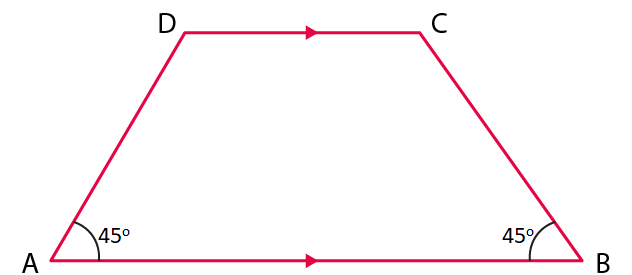We know that,

Angles opposite to each other in quadrilateral are supplementary.

Then, we have,

∠A + ∠C = 180º

45o + ∠C = 180º

∠C = 180o – 45o

∠C = 135o

Similarly,

We have,

∠B + ∠D = 180º

45o + ∠D = 180º

∠D = 180o – 45o

∠D = 135o

3. The angle between two altitudes of a parallelogram through the vertex of an obtuse angle of the parallelogram is 60º. Find the angles of the parallelogram.

Solution: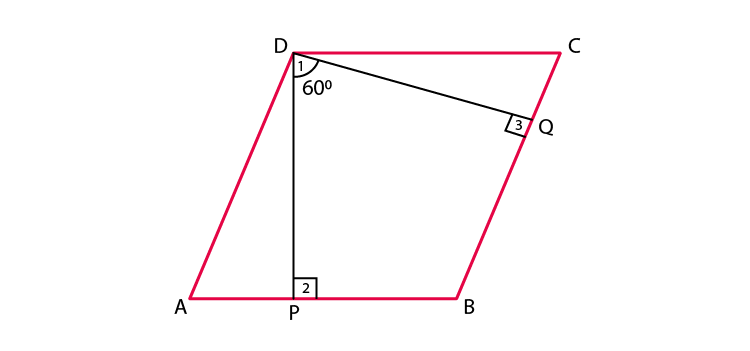According to the question,

ABCD is parallelogram,

DP ⊥ AB

DQ ⊥ BC.

∠PDQ = 60o

Using angle sum property of a quadrilateral,

We have,

∠PDQ + ∠Q + ∠P + ∠B = 360o

60o + 90o + 90o + ∠B = 360o

240o + ∠B = 360o

∠B = 360o – 240o

∠B = 120o

Since, opposite angles in parallelogram are equal,

We have,

∠B = ∠D = 120o

Since, opposite sides are parallel in parallelogram,

We have,

AB||CD

Also, since sum of adjacent interior angles is 180o,

We have,

∠B + ∠C = 180o

120o + ∠C = 180o

∠C = 180o – 120o

∠C = 60o

Since, opposite angles in parallelogram are equal,

We have,

∠C = ∠A = 60o

4. ABCD is a rhombus in which altitude from D to side AB bisects AB. Find the angles of the rhombus.

Solution: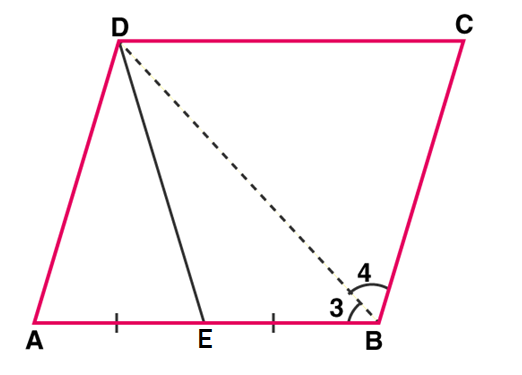According to the question,

We have,

ABCD is a rhombus.

DE is the altitude on AB then AE = EB.

In ΔAED and ΔBED,

We have,

DE = DE (common line)

∠AED = ∠BED (right angle)

AE = EB (DE is an altitude)

∴ ΔAED ≅ ΔBED by SAS property.

∴ AD = BD (by C.P.C.T)

But AD = AB (sides of rhombus are equal)

⇒ AD = AB = BD

∴ ABD is an equilateral triangle.

∴ ∠A = 60o

Since, opposite angles of rhombus are equal, we get,

⇒ ∠A = ∠C = 60o

We also know that,

Sum of adjacent angles of a rhombus = supplementary.

So,

∠ABC + ∠BCD = 180o

∠ABC + 60o = 180o

∠ABC = 180o – 60o = 120o

Since, opposite angles of rhombus are equal, we get,

Hence, Angles of rhombus are:

∠A = 60o, ∠C = 60o, ∠B = 120o, ∠D = 120o

5. E and F are points on diagonal AC of a parallelogram ABCD such that AE = CF. Show that BFDE is a parallelogram.

Solution: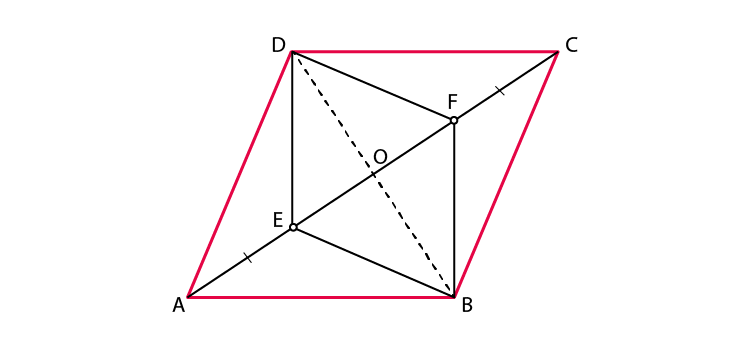Construction:

Join BD, meeting AC at O.

According to the question,

Since diagonals of a parallelogram bisect each other,

We get,

OA = OC and OD = OB.

And,

OA = OC and AE = CF,

OA – AE = OC – CF

OE = OF

So, BFDE is a quadrilateral whose diagonals bisect each other.

Hence, BFDE is a parallelogram.

Exercise 8.4 Page No: 82

1. A square is inscribed in an isosceles right triangle so that the square and the triangle have one angle common. Show that the vertex of the square opposite the vertex of the common angle bisects the hypotenuse.

Solution: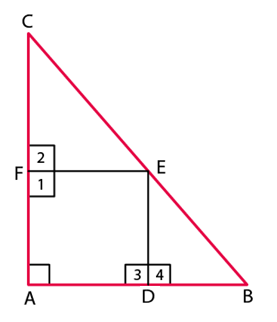According to the question,

ΔABC with ∠A = 90o and

Since, ABC is an isosceles triangle,

We get,

AB = AC …(i)

Let ADEF be the square inscribed in the isosceles triangle ABC.

Then, we have,

Subtracting equation (ii) from (i),

AB – AD = AC – AF

BD = CF

Now,

Considering ΔCFE and ΔEDB,

BD = CF

DE = EF

∠CFE = ∠EDB = 90o (Since, they are the side of a square)

ΔCEF ~ ΔBED (By SAS criteria)

Hence, CE = BE

Therefore, vertex E of the square bisect the hypotenuse BC.

2. In a parallelogram ABCD, AB = 10 cm and AD = 6 cm. The bisector of ∠A meets DC in E. AE and BC produced meet at F. Find the length of CF.

Solution: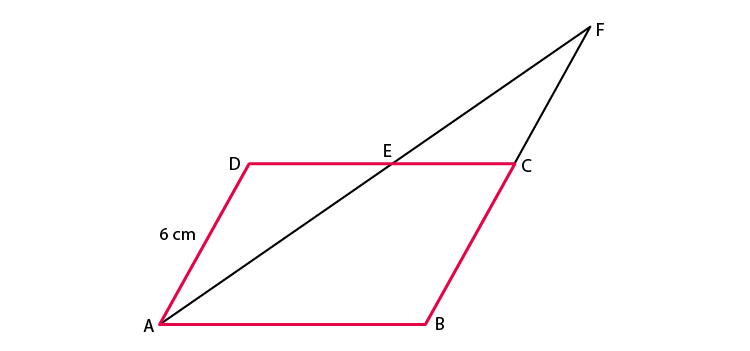According to the question,

We have,

ABCD is a parallelogram

AB = 10 cm

The bisector of ∠A meets DC at E.

AE and BC produced meet at F.

Since, AF bisects ∠A,

We get,

∠EAD = ∠EFB … (2) [Alternate angles]

From equations (1) and (2),

We get,

∠BAE = ∠EFB

Since sides opposite to equal angles are equal,

We get,

BF = AB

Here, AB = 10 cm

So, BF = 10 cm

⇒ BC + CF = 10 cm

6 cm + CF = 10 cm [BC = AD = 6 cm, opposite sides of a parallelogram]

⇒ CF = 10 – 6 cm = 4 cm

⇒ CF = 4 cm

3. P, Q, R and S are respectively the mid-points of the sides AB, BC, CD and DA of a quadrilateral ABCD in which AC = BD. Prove that PQRS is a rhombus.

Solution: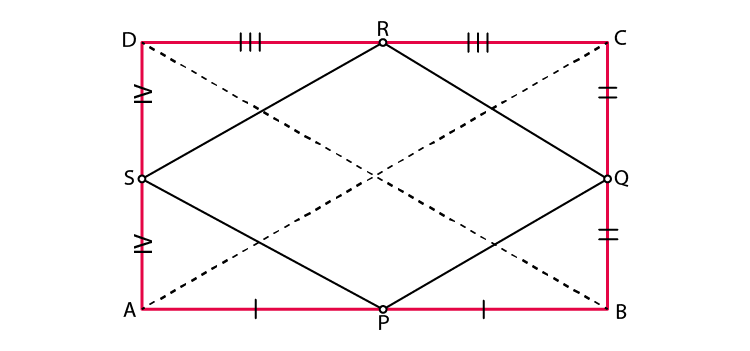According to the question,

We have,

P is the mid-point of the sides AB

Q is the mid-point of the sides BC

R is the mid-point of the sides CD

S is the mid-point of the sides DA

Also, we know that,

AC = BD.

SR = ½ AC

And, SR||AC

In ΔABC, by mid-point theorem,

PQ = ½ AC

And, PQ||AC

Hence, SR = PQ = ½ AC

Similarly,

In ΔBCD, by mid-point theorem,

RQ = ½ BD

And, RQ||BD

SP = ½ BD

And, SP||BD

So, we get,

SP = RQ = ½ BD = ½ AC

Then,

SR = PQ = SP = RQ

Hence, PQRS is a rhombus.

4. P, Q, R and S are respectively the mid-points of the sides AB, BC, CD and DA of a quadrilateral ABCD such that AC ⊥ BD. Prove that PQRS is a rectangle.

Solution: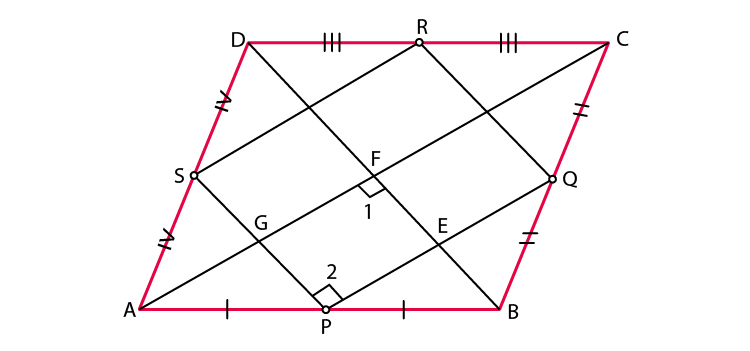According to the question,

We have,

P is the mid-point of the sides AB

Q is the mid-point of the sides BC

R is the mid-point of the sides CD

S is the mid-point of the sides DA

Also,

AC ⊥ BD

∠COD = ∠AOD = ∠AOB = ∠COB = 90o

SR = ½ AC

And, SR||AC

In ΔABC, by mid-point theorem,

PQ = ½ AC

And, PQ||AC

So, we have,

PQ||SR and SR = PQ = ½ AC

Similarly,

SP||RQ and SP = RQ = ½ BD

OE||FR and OF||ER

So, we get,

∠EOF = ∠ERF = 90o

Hence, PQRS is a rectangle.

5. P, Q, R and S are respectively the mid-points of sides AB, BC, CD and DA of quadrilateral ABCD in which AC = BD and AC ⊥ BD. Prove that PQRS is a square.

Solution:

According to the question,

We have,

P is the mid-point of the sides AB

Q is the mid-point of the sides BC

R is the mid-point of the sides CD

S is the mid-point of the sides DA

Also,

AC ⊥ BD

And AC = BD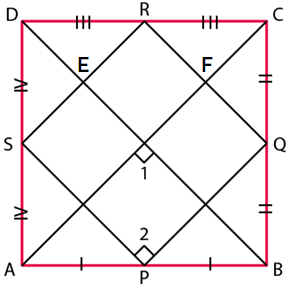SR = ½ AC

And, SR||AC

In ΔABC, by mid-point theorem,

PQ = ½ AC

And, PQ||AC

So, we have,

PQ||SR and PQ = SR = ½ AC

Now, in ΔABD, by mid-point theorem,

SP||BD and SP = ½ BD = ½ AC

In ΔBCD, by mid-point theorem,

RQ||BD and RQ = ½ BD = ½ AC

SP = RQ = ½ AC

PQ = SR = SP = RQ

Thus, we get that,

All four sides are equal.

OE||FR, OF||ER

∠EOF = ∠ERF = 90o (Opposite angles of parallelogram)

∠QRS = 90o

Hence, PQRS is a square.

6. A diagonal of a parallelogram bisects one of its angles. Show that it is a rhombus.

Solution:

Let the parallelogram be = ABCD

Diagonal AC bisect ∠A.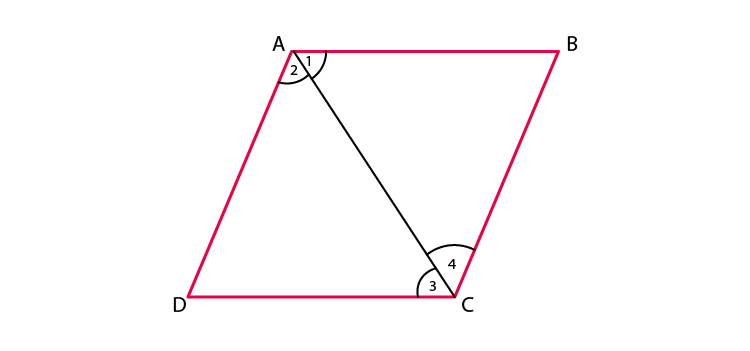Now,

AB||CD and AC is a transversal.

∠CAB = ∠ACD

Again, AD||BC and AC is a transversal.

∠DAC = ∠ACB

Now,

∠A = ∠C

½ ∠A = ½ ∠C

∠DAC = ∠DCA

But, AB = CD and AD = BC (Opposite sides of parallelograms)

AB = BC = CD = AD

Thus, ABCD is a rhombus.

7. P and Q are the mid-points of the opposite sides AB and CD of a parallelogram ABCD. AQ intersects DP at S and BQ intersects CP at R. Show that PRQS is a parallelogram.

Solution:

According to the question,

Q is the midpoint of AB

P is the midpoint of CD

Now,

AB||CD,

Also,

AP||QC

And, AB = DC

½ AB = ½ DC

AP = QC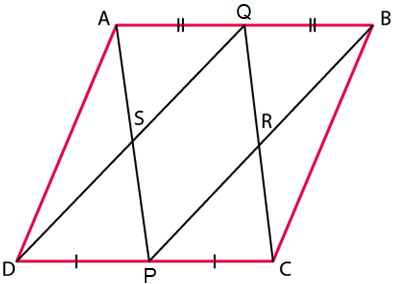Now,

AP||QC and AP = QC

APCQ is a parallelogram.

AQ||PC or SQ||PR

Again,

AB||DC means ½ AB = ½ DC

BP = QD

Now, BP||QD and BP = QD

BPDQ is a parallelogram

So, PD||BQ or PS||QR

Thus, SQ||RP and PS||QR

PQRS is a parallelogram.

8. ABCD is a quadrilateral in which AB || DC and AD = BC. Prove that ∠A = ∠B and ∠C = ∠D.

Solution: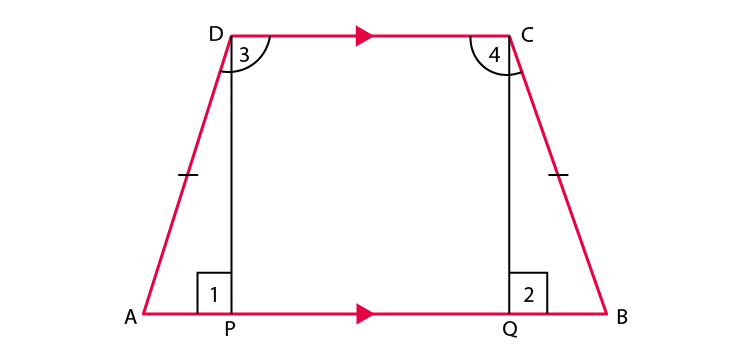According to the question,

We have,

To prove: ∠A = ∠B and ∠C = ∠D.

Construction: Draw DP ⊥ AB and CQ ⊥ AB.

Proof: In ΔAPD and ΔBQC,

Since ∠1 and ∠2 are equal to 90o

∠1 = ∠2

Distance between parallel line,

AB = BC [Given]

By RHS criterion of congruence,

We have

ΔAPD ≅ ΔBQC [CPCT]

∠A = ∠B

Now, DC||AB

Since, sum of consecutive interior angles is 180o

∠A+∠3 =180 …(1)

And,

∠B +∠4 =180 …(2)

From equations (1) and (2),

We get

∠A + ∠3 = ∠B + ∠4

Since, ∠A = ∠B,

We have,

⇒ ∠3 = ∠4

⇒ ∠C = ∠D

Hence, proved.

9. In Fig. 8.11, AB || DE, AB = DE, AC || DF and AC = DF. Prove that BC || EF and BC = EF.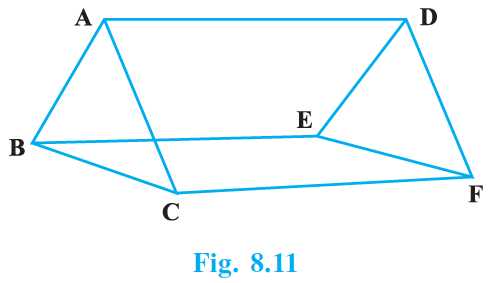Solution: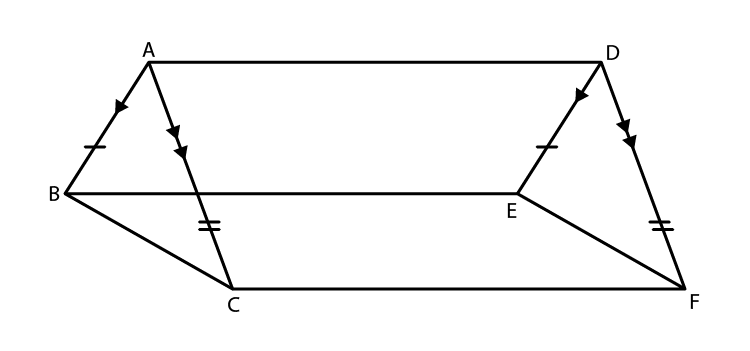According to the question,

We have,

AB||DE and AB = DE

ABED is a parallelogram.

We have,

AC||FD and AC = FD

ACFD is a parallelogram.

AD = BE = CF and CF||BE

BE = CF and BE||CF.

BCFE is a parallelogram.

BC = EF and BC||EF

Hence proved.

Our experts have prepared these Exemplar Solutions in such a way that students can easily resolve all the difficulties faced while solving NCERT Book exercise questions for Class 9 Chapter 8. These solved questions can be considered as reference tools for them. Apart from these, there are NCERT Solutions, notes, and previous year question papers available here with us for standard 9th students to make them ready for final exams. Practice question papers and sample papers to understand the question pattern for this chapter and marks carried by it in final exams.

Keep reading with us and get exemplars for all the chapters of Class 9 Maths subjects. Download the BYJU’S app to get personalized video content and learn the concepts of Maths such as quadrilaterals in an easy way.

## Frequently Asked Questions on NCERT Solutions for Class 11 Maths Chapter 8

### Explain the concepts covered in Chapter 8 of NCERT Exemplar Solutions for Class 9 Maths.

The concepts covered in Chapter 8 of NCERT Exemplar Solutions for Class 9 Maths are
1. Angle sum property of quadrilaterals and it’s proof
2. Types of quadrilaterals such as Trapezium, Parallelogram, Square, Rectangle, Rhombus, and Kite.
3. Properties of parallelogram and condition for a quadrilateral to be a parallelogram
4. Problems based on mid-point theorem
Students can now study and be updated about the latest syllabus of the CBSE board using the NCERT Exemplar Solutions which are available in PDF format.

### Will the NCERT Exemplar Solutions for Class 9 Maths Chapter 8 help students to understand the concepts which are important from the exam perspective?

In order to understand the expansion procedure, students can refer to the examples which are present in the NCERT textbook before solving the exercise wise problems. Each problem in the solutions is solved in a stepwise manner to help students in understanding the concepts in a better way. By using the solutions PDF, students will be well versed with the method of solving these equations and score well in the exam.

### Explain the concept of the midpoint theorem covered in the NCERT Exemplar Solutions for Class 9 Maths Chapter 8?

The midpoint theorem states that “The line segment in a triangle joining the midpoint of two sides of the triangle is said to be parallel to its third side and is also half of the length of the third side.” Question paper in the annual exam would target the chapters which are simple for the students but tricky to solve. For this purpose, students should go through the NCERT Solutions if they aspire to score good marks.